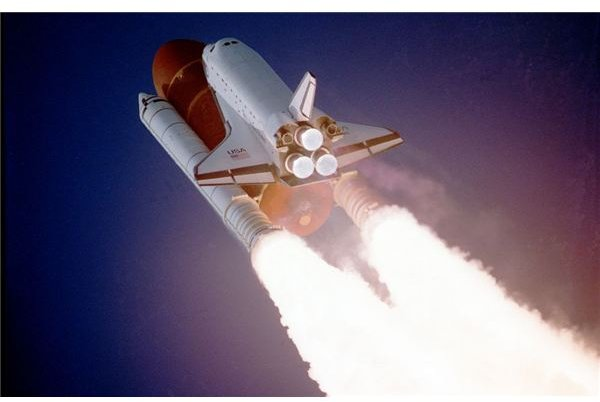# Newton's Laws Concerning Gravitation and How They Apply to Astronomy

Page content

## Newton’s Contributions

Sir Isaac Newton, historically one of the most inspired of scientists, is famous for his three laws relating to force, mass, momentum, and acceleration. Although we’re living in times not entirely defined by classical mechanics, those laws still apply and are fundamental in the field of astronomy.

## Newton’s First, Second, and Third Laws of Motion

Newton’s First Law: This is called the “law of inertia.” A body remains in a constant state of motion or at rest unless acted upon by an external force. In other words, if all forces exerted on an object add up to zero, its state of motion will remain constant; its acceleration will be zero.

Newton’s Second Law: A force applied to an object produces a time rate of change of its linear momentum.

In mathematical jargon, the force is equal to the derivative of the linear momentum with respect to time.

F = d(mv)/dt

Since by classical mechanics mass is constant, for most purposes the expression may be simplified,

F = ma

Newton’s Third Law: For every action, there is an equal and opposite reaction. This tells us that if a force, F, is applied to an object, then that object responds with an equal force of opposite direction, -F. Combined with the second law, its equivalent is,

m1a1 = -m2a2

As an example, a hunter pulls the trigger that fires a bullet from a gun. A reactive force is very real; the gun recoils. In the case of a rocket, the fuel ignites and pushes the rocket forward. The rocket pushes back and the exhausted products exit from the rear. In this instance, both the second and third laws obviously apply.

If an applied force is constant, as in the case of the rocket and not of the bullet, acceleration is constant. The Earth is always under acceleration while orbiting the Sun, the force must be continuous - in this instance the force is the gravitational force of the Sun.

## Newton’s Law of Gravitation

For two masses, m1 and m2, with a distance r between them,

F = Gm1m2/r2,

Where G is the gravitational constant, and is equal to: 6.67 x 10-11 m3/kg-sec2.

How can this expression be applied to “free fall?”

Since, F = ma, if we make m₂ = M, the mass of the earth, then the acceleration due to Earth’s gravity is:

g = GM/ r2.

Knowing either the mass of the body or its gravitational acceleration, the other variable can be calculated.

## Application

There are at least two applications of Newton’s laws of gravitation to astronomy-related science. One application is to man-made space vehicles. The other application is to the heavenly bodies, themselves.As an example of the latter, Newton’s law of gravitation can be combined with his second law and applied to a comet passing by a star. The comet is accelerated. This is because the star is very massive in comparison with the comet. Even so, the comet exerts a calculable force upon the star, theoretically moving it!

What sort of numbers might we be talking about? Assume the mass m1 of the comet is 2 x 1014kg, the mass m2 of the star is 2 × 1030 kg, and the distance between them r is 1.0 x 1010m.Then

F = Gm1m2/r2

F = (6.67300 × 10-11 m3 /kg-s2) x (2 x 1014kg) x (2 × 1030 kg) / (1.0 x 1010m)2

F = 2.67 x 1014kg-m/s2.

Now,

F =ma, or a = F/m.

For the comet, the acceleration received is,

a = 2.67 x 1014kg-m/s2 / 2 x 1014kg, so

a comet = 1.34 m/s2.

For the star,

a = 2.67 x 1014kg-m/s2 / 2 × 1030 kg, so

a star = 1.34 x 10-16m/s2.

Clearly, the star is nearly unaccelerated, while the comet receives a considerable “kick.”

## Gravitational Perturbation

Envisioning the orbit of a planet about the sun as a two-body problem, although an excellent first-order approximation, is not totally accurate. The two reasons it generally works are that in Newton’s law of gravitation, the sun’s mass and the mass of the planets are large, and secondly, other bodies of sizeable mass are very distant from the solar system. Since distance is squared in Newton’s equation, other stars exert negligible influence.

One exception led to a startling discovery in the middle 1800’s.

The simple two-body calculations didn’t work well for Uranus. Clearly some perturbation was interfering with the calculations. That something proved to be the planet Neptune.

Thus, although there are newer and more complicated mathematical systems than those proposed by Newton, the equations attributed to him still bear relevancy to astronomical sciences today.